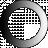# American Dictionary of the English Language

Dictionary Search

### Cube

CUBE, noun [Gr., Latin , a die or cube; to set or throw down, that which is set or laid, a solid mass.]

1. In geometry, a regular solid body, with six equal sides, and containing equal angles.

2. In arithmetic, the product of a number multiplied into itself, and that product multiplied into the same number; or it is formed by multiplying any number twice by itself; as, 4 times 4 = 16, and 16 times 4 = 64, the cube of 4.

The law of the planets is, that the squares of the times of their revolutions are in proportion to the cubes of their mean distances.

CUBE root, is the number or quantity, which, multiplied into itself, and then into the product, produces the cube; or which, twice multiplied into itself, produces the number of which it is the root; thus, 3 is the cube root or side of 27, for 3 times 3 = 9, and 3 times 9 = 27.